# Scheduling and Timetables in Airflow

One of the fundamental features of Apache Airflow is the ability to schedule jobs. Historically, Airflow users could schedule their DAGs by specifying a `schedule` with a cron expression, a timedelta object, or a preset Airflow schedule.

Timetables, released in Airflow 2.2, brought new flexibility to scheduling. Timetables allow users to create their own custom schedules using Python, effectively eliminating the limitations of cron. With timetables, you can now schedule DAGs to run at any time for any use case.

Additionally, Airflow 2.4 introduced datasets and the ability to schedule your DAGs on updates to a dataset rather than a time-based schedule. A more in-depth explanation on these features can be found in the Datasets and Data Driven Scheduling in Airflow guide.

In this guide, we’ll walk through Airflow scheduling concepts and the different ways you can schedule a DAG with a focus on timetables. For additional instructions check out our Scheduling in Airflow webinar.

Note: All code in this guide can be found in this repo.

## Assumed knowledge

To get the most out of this guide, you should have knowledge of:

## Scheduling concepts

There are a couple of terms and parameters in Airflow that are important to understand related to scheduling.

• Data Interval: The data interval is a property of each DAG run that represents the period of data that each task should operate on. For example, for a DAG scheduled hourly each data interval will begin at the top of the hour (minute 0) and end at the close of the hour (minute 59). The DAG run is typically executed at the end of the data interval, depending on whether your DAG’s schedule has “gaps” in it.
• Logical Date: The logical date of a DAG run is the same as the start of the data interval. It does not represent when the DAG will actually be executed. Prior to Airflow 2.2, this was referred to as the execution date.
• Timetable: The timetable is a property of a DAG that dictates the data interval and logical date for each DAG run (i.e. it determines when a DAG will be scheduled).
• Run After: The earliest time the DAG can be scheduled. This date is shown in the Airflow UI, and may be the same as the end of the data interval depending on your DAG’s timetable.
• Backfilling and Catchup: We won’t cover these concepts in depth here, but they can be related to scheduling. We recommend reading the Apache Airflow documentation on them to understand how they work and whether they’re relevant for your use case.

Note: In this guide we do not cover the `execution_date` concept, which has been deprecated as of Airflow 2.2. If you are using older versions of Airflow, review this doc for more on `execution_date`.

### Parameters

The following parameters are derived from the concepts described above and are important for ensuring your DAG runs at the correct time.

• `data_interval_start`: A datetime object defining the start date and time of the data interval. A DAG’s timetable will return this parameter for each DAG run. This parameter is either created automatically by Airflow, or can be specified by the user when implementing a custom timetable.
• `data_interval_end`: A datetime object defining the end date and time of the data interval. A DAG’s timetable will return this parameter for each DAG run. This parameter is either created automatically by Airflow, or can be specified by the user when implementing a custom timetable.
• `schedule`: A parameter that can be set at the DAG level to define when that DAG will be run. It accepts cron expressions, timedelta objects, timetables, and lists of datasets.
• `start_date`: The first date your DAG will be executed. This parameter is required for your DAG to be scheduled by Airflow.
• `end_date`: The last date your DAG will be executed. This parameter is optional.

Note In Airflow 2.3 or older, the `schedule` parameter is called `schedule_interval` and only accepts cron expressions or timedelta objects. Additionally, timetables have to be passed using the `timetable` parameter, which is deprecated in Airflow 2.4+. In versions of Airflow 2.2 and earlier, specifying `schedule_interval` is the only way to define a DAG’s schedule.

### Example

As a simple example of how these concepts work together, say we have a DAG that is scheduled to run every 5 minutes. Looking at the most recent DAG run, the logical date is `2022-08-28 22:37:33` (shown below Run next to the DAG name in the UI), which is the same as the Data interval start shown in the bottom right corner of in the screenshot below. The logical date is also the timestamp that will be incorporated into the Run ID of the DAG run which is how the DAG run is identified in the Airflow metadata database. The Data interval end is 5 minutes later.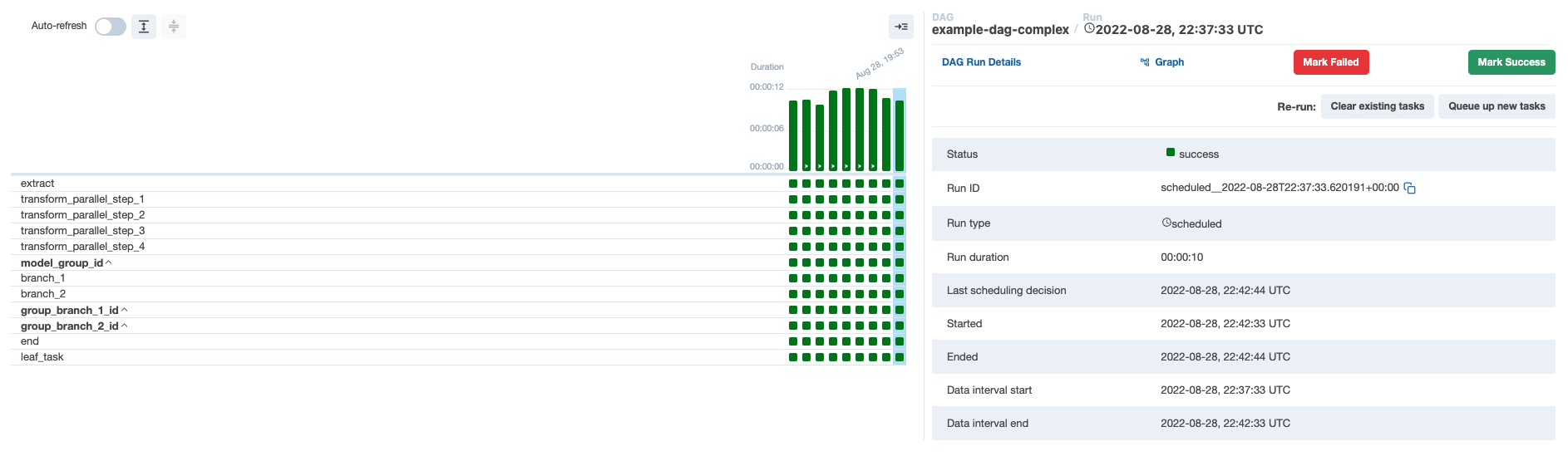If we look at the next DAG run in the UI, the logical date is `2022-08-28 22:42:33`, which is shown as the Next Run timestamp in the UI. This is 5 minutes after the previous logical date, and the same as the Data interval end of the last DAG run because there are no gaps in the schedule. If we hover over Next Run, we can see that Run After, which is the date and time that the next DAG run will actually start, is also the same as the next DAG run’s Data interval end: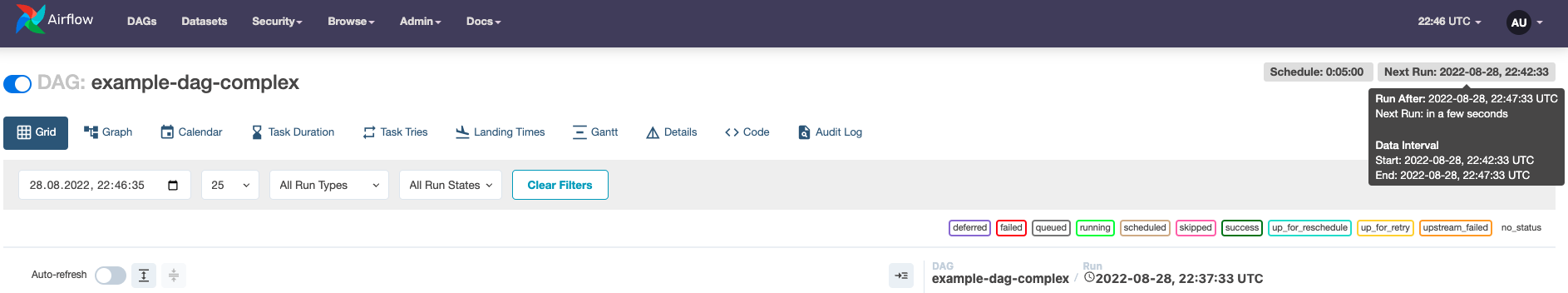In summary we’ve described 2 DAG runs:

• DAG run 1 with the Run ID `scheduled__2022-08-28T22:37:33.620191+00:00` has a logical date of `2022-08-28 22:37:33`, a Data interval start of `2022-08-28 22:37:33` and a Data interval end of `2022-08-28 22:42:33`. This DAG run will actually start at `2022-08-28 22:42:33`.
• DAG run 2 with the Run ID `scheduled__2022-08-28T22:42:33.617231+00:00` has a logical date of `2022-08-28 22:42:33` (shown as Next Run in the UI in the second screenshot), a Data interval start of `2022-08-28 22:42:33` and a Data interval end of `2022-08-28 22:47:33`. This DAG run will actually start at `2022-08-28 22:47:33` (shown as Run After in the UI in the second screenshot).

In the sections below, we’ll walk through how to use cron-based schedule, timetables, or datasets to schedule your DAG.

## Cron-based schedules

For pipelines with simple scheduling needs, you can define a `schedule` in your DAG using:

• A cron expression.
• A cron preset.
• A timedelta object.

### Setting a cron-based schedule

#### Cron expressions

You can pass any cron expression as a string to the `schedule` parameter in your DAG. For example, if you want to schedule your DAG at 4:05 AM every day, you would use `schedule='5 4 * * *'`.

If you need help creating the correct cron expression, crontab guru is a great resource.

#### Cron presets

Airflow can utilize cron presets for common, basic schedules.

For example, `schedule='@hourly'` will schedule the DAG to run at the beginning of every hour. For the full list of presets, check out the Airflow documentation. If your DAG does not need to run on a schedule and will only be triggered manually or externally triggered by another process, you can set `schedule=None`.

#### Timedelta objects

If you want to schedule your DAG on a particular cadence (hourly, every 5 minutes, etc.) rather than at a specific time, you can pass a `timedelta` object imported from the `datetime` package to the `schedule` parameter. For example, `schedule=timedelta(minutes=30)` will run the DAG every thirty minutes, and `schedule=timedelta(days=1)` will run the DAG every day.

Note: Do not make your DAG’s schedule dynamic (e.g. `datetime.now()`)! This will cause an error in the Scheduler.

### Cron-based schedules & the logical date

Airflow was originally developed for ETL under the expectation that data is constantly flowing in from some source and then will be summarized on a regular interval. If you want to summarize Monday’s data, you can only do it after Monday is over (Tuesday at 12:01 AM). However, this assumption has turned out to be ill-suited to the many other things Airflow is being used for now. This discrepancy is what led to Timetables, which were introduced in Airflow 2.2.

Each DAG run therefore has a `logical_date` that is separate from the time that the DAG run is expected to begin (`logical_date` was called `execution_date` before Airflow 2.2). A DAG run is not actually allowed to run until the `logical_date` for the following DAG run has passed. So if you are running a daily DAG, Monday’s DAG run will not actually execute until Tuesday. In this example, the `logical_date` would be Monday 12:01 AM, even though the DAG run will not actually begin until Tuesday 12:01 AM.

If you want to pass a timestamp to the DAG run that represents “the earliest time at which this DAG run could have started”, use `{{ next_ds }}` from the jinja templating macros.

Note: It is best practice to make each DAG run idempotent (able to be re-run without changing the result) which precludes using `datetime.now()`.

### Limitations of cron-based schedules

The relationship between a DAG’s `schedule` and its `logical_date` leads to particularly unintuitive results when the spacing between DAG runs is irregular. The most common example of irregular spacing is when DAGs run only during business days (Mon-Fri). In this case, the DAG run with an `logical_date` of Friday will not run until Monday, even though all of Friday’s data will be available on Saturday. This means that a DAG whose desired behavior is to summarize results at the end of each business day actually cannot be set using only the `schedule`. In versions of Airflow prior to 2.2, one must instead schedule the DAG to run every day (including the weekend) and include logic in the DAG itself to skip all tasks for days on which the DAG doesn’t really need to run.

In addition, it is difficult or impossible to implement situations like the following using a traditional schedule:

• Schedule a DAG at different times on different days, like 2pm on Thursdays and 4pm on Saturdays.
• Schedule a DAG daily except for holidays.
• Schedule a DAG at multiple times daily with uneven intervals (e.g. 1pm and 4:30pm).

In the next section, we’ll describe how these limitations were addressed in Airflow 2.2 with the introduction of timetables.

## Timetables

Timetables, introduced in Airflow 2.2, address the limitations of cron expressions and timedelta objects by allowing users to define their own schedules in Python code. All DAG schedules are ultimately determined by their internal timetable and if a cron expression or timedelta object is not sufficient for your use case, you can define your own.

Custom timetables can be registered as part of an Airflow plugin. They must be a subclass of `Timetable`, and they should contain the following methods, both of which return a `DataInterval` with a start and an end:

• `next_dagrun_info`: Returns the data interval for the DAG’s regular schedule
• `infer_manual_data_interval`: Returns the data interval when the DAG is manually triggered

Below we’ll show an example of implementing these methods in a custom timetable.

### Example custom timetable

For this implementation, let’s run our DAG at 6:00 and 16:30. Because this schedule has run times with differing hours and minutes, it can’t be represented by a single cron expression. But we can easily implement this schedule with a custom timetable!

To start, we need to define the `next_dagrun_info` and `infer_manual_data_interval` methods. Before diving into the code, it’s helpful to think through what the data intervals will be for the schedule we want. Remember that the time the DAG runs (`run_after`) should be the end of the data interval since our interval has no gaps. So in this case, for a DAG that we want to run at 6:00 and 16:30, we have two different alternating intervals:

• Run at 6:00: Data interval is from 16:30 on the previous day to 6:00 on the current day
• Run at 16:30: Data interval is from 6:00 to 16:30 on the current day

With that in mind, first we’ll define `next_dagrun_info`. This method provides Airflow with the logic to calculate the data interval for scheduled runs. It also contains logic to handle the DAG’s `start_date`, `end_date`, and `catchup` parameters. To implement the logic in this method, we use the Pendulum package, which makes dealing with dates and times simple. The method looks like this:

``````def next_dagrun_info(
self,
*,
last_automated_data_interval: Optional[DataInterval],
restriction: TimeRestriction,
) -> Optional[DagRunInfo]:
if last_automated_data_interval is not None:  # There was a previous run on the regular schedule.
last_start = last_automated_data_interval.start
delta = timedelta(days=1)
if last_start.hour == 6: # If previous period started at 6:00, next period will start at 16:30 and end at 6:00 following day
next_start = last_start.set(hour=16, minute=30).replace(tzinfo=UTC)
next_end = (last_start+delta).replace(tzinfo=UTC)
else: # If previous period started at 16:30, next period will start at 6:00 next day and end at 16:30
next_start = (last_start+delta).set(hour=6, minute=0).replace(tzinfo=UTC)
next_end = (last_start+delta).replace(tzinfo=UTC)
else:  # This is the first ever run on the regular schedule. First data interval will always start at 6:00 and end at 16:30
next_start = restriction.earliest
if next_start is None:  # No start_date. Don't schedule.
return None
if not restriction.catchup: # If the DAG has catchup=False, today is the earliest to consider.
next_start = max(next_start, DateTime.combine(Date.today(), Time.min).replace(tzinfo=UTC))
next_start = next_start.set(hour=6, minute=0).replace(tzinfo=UTC)
next_end = next_start.set(hour=16, minute=30).replace(tzinfo=UTC)
if restriction.latest is not None and next_start > restriction.latest:
return None  # Over the DAG's scheduled end; don't schedule.
return DagRunInfo.interval(start=next_start, end=next_end)``````

Walking through the logic, this code is equivalent to:

• If there was a previous run for the DAG:
• If the previous DAG run started at 6:00, then the next DAG run should start at 16:30 and end at 6:00 the next day.
• If the previous DAG run started at 16:30, then the DAG run should start at 6:00 the next day and end at 16:30 the next day.
• If it is the first run of the DAG:
• Check for a start date. If there isn’t one, the DAG can’t be scheduled.
• Check if `catchup=False`. If so, the earliest date to consider should be the current date. Otherwise it is the DAG’s start date.
• We’re mandating that the first DAG run should always start at 6:00, so update the time of the interval start to 6:00 and the end to 16:30.
• If the DAG has an end date, do not schedule the DAG after that date has passed.

Then we define the data interval for manually triggered DAG runs by defining the `infer_manual_data_interval` method. The code looks like this:

``````def infer_manual_data_interval(self, run_after: DateTime) -> DataInterval:
delta = timedelta(days=1)
# If time is between 6:00 and 16:30, period ends at 6am and starts at 16:30 previous day
if run_after >= run_after.set(hour=6, minute=0) and run_after <= run_after.set(hour=16, minute=30):
start = (run_after-delta).set(hour=16, minute=30, second=0).replace(tzinfo=UTC)
end = run_after.set(hour=6, minute=0, second=0).replace(tzinfo=UTC)
# If time is after 16:30 but before midnight, period is between 6:00 and 16:30 the same day
elif run_after >= run_after.set(hour=16, minute=30) and run_after.hour <= 23:
start = run_after.set(hour=6, minute=0, second=0).replace(tzinfo=UTC)
end = run_after.set(hour=16, minute=30, second=0).replace(tzinfo=UTC)
# If time is after midnight but before 6:00, period is between 6:00 and 16:30 the previous day
else:
start = (run_after-delta).set(hour=6, minute=0).replace(tzinfo=UTC)
end = (run_after-delta).set(hour=16, minute=30).replace(tzinfo=UTC)
return DataInterval(start=start, end=end)``````

This method figures out what the most recent complete data interval is based on the current time. There are three scenarios:

• The current time is between 6:00 and 16:30: In this case, the data interval is from 16:30 the previous day to 6:00 the current day.
• The current time is after 16:30 but before midnight: In this case, the data interval is from 6:00 to 16:30 the current day.
• The current time is after midnight but before 6:00: In this case, the data interval is from 6:00 to 16:30 the previous day.

We need to account for time periods in the same timeframe (6:00 to 16:30) on different days than the day that the DAG is triggered, which requires three sets of logic. When defining custom timetables, always keep in mind what the last complete data interval should be based on when the DAG should run.

Now we can take those two methods and combine them into a `Timetable` class which will make up our Airflow plugin. The full custom timetable plugin is below:

``````from datetime import timedelta
from typing import Optional
from pendulum import Date, DateTime, Time, timezone

from airflow.plugins_manager import AirflowPlugin
from airflow.timetables.base import DagRunInfo, DataInterval, TimeRestriction, Timetable

UTC = timezone("UTC")

class UnevenIntervalsTimetable(Timetable):

def infer_manual_data_interval(self, run_after: DateTime) -> DataInterval:
delta = timedelta(days=1)
# If time is between 6:00 and 16:30, period ends at 6am and starts at 16:30 previous day
if run_after >= run_after.set(hour=6, minute=0) and run_after <= run_after.set(hour=16, minute=30):
start = (run_after-delta).set(hour=16, minute=30, second=0).replace(tzinfo=UTC)
end = run_after.set(hour=6, minute=0, second=0).replace(tzinfo=UTC)
# If time is after 16:30 but before midnight, period is between 6:00 and 16:30 the same day
elif run_after >= run_after.set(hour=16, minute=30) and run_after.hour <= 23:
start = run_after.set(hour=6, minute=0, second=0).replace(tzinfo=UTC)
end = run_after.set(hour=16, minute=30, second=0).replace(tzinfo=UTC)
# If time is after midnight but before 6:00, period is between 6:00 and 16:30 the previous day
else:
start = (run_after-delta).set(hour=6, minute=0).replace(tzinfo=UTC)
end = (run_after-delta).set(hour=16, minute=30).replace(tzinfo=UTC)
return DataInterval(start=start, end=end)

def next_dagrun_info(
self,
*,
last_automated_data_interval: Optional[DataInterval],
restriction: TimeRestriction,
) -> Optional[DagRunInfo]:
if last_automated_data_interval is not None:  # There was a previous run on the regular schedule.
last_start = last_automated_data_interval.start
delta = timedelta(days=1)
if last_start.hour == 6: # If previous period started at 6:00, next period will start at 16:30 and end at 6:00 following day
next_start = last_start.set(hour=16, minute=30).replace(tzinfo=UTC)
next_end = (last_start+delta).replace(tzinfo=UTC)
else: # If previous period started at 14:30, next period will start at 6:00 next day and end at 14:30
next_start = (last_start+delta).set(hour=6, minute=0).replace(tzinfo=UTC)
next_end = (last_start+delta).replace(tzinfo=UTC)
else:  # This is the first ever run on the regular schedule. First data interval will always start at 6:00 and end at 16:30
next_start = restriction.earliest
if next_start is None:  # No start_date. Don't schedule.
return None
if not restriction.catchup: # If the DAG has catchup=False, today is the earliest to consider.
next_start = max(next_start, DateTime.combine(Date.today(), Time.min).replace(tzinfo=UTC))
next_start = next_start.set(hour=6, minute=0).replace(tzinfo=UTC)
next_end = next_start.set(hour=16, minute=30).replace(tzinfo=UTC)
if restriction.latest is not None and next_start > restriction.latest:
return None  # Over the DAG's scheduled end; don't schedule.
return DagRunInfo.interval(start=next_start, end=next_end)

class UnevenIntervalsTimetablePlugin(AirflowPlugin):
name = "uneven_intervals_timetable_plugin"
timetables = [UnevenIntervalsTimetable]``````

Note that because timetables are plugins, you will need to restart the Airflow Scheduler and Webserver after adding or updating them.

In the DAG, we can then import the custom timetable plugin and use it to schedule the DAG by setting the `timetable` parameter:

``````from uneven_intervals_timetable import UnevenIntervalsTimetable

with DAG(
dag_id="example_timetable_dag",
start_date=datetime(2021, 10, 9),
max_active_runs=1,
timetable=UnevenIntervalsTimetable(),
default_args={
"retries": 1,
"retry_delay": timedelta(minutes=3),
},
catchup=True
) as dag:``````

Looking at the Tree View in the UI, we can see that this DAG has run twice per day at 6:00 and 16:30 since the start date of 2021-10-09.The next scheduled run is for the interval starting on 2021-10-12 at 16:30 and ending the following day at 6:00. This run will be triggered at the end of the data interval, so after 2021-10-13 6:00.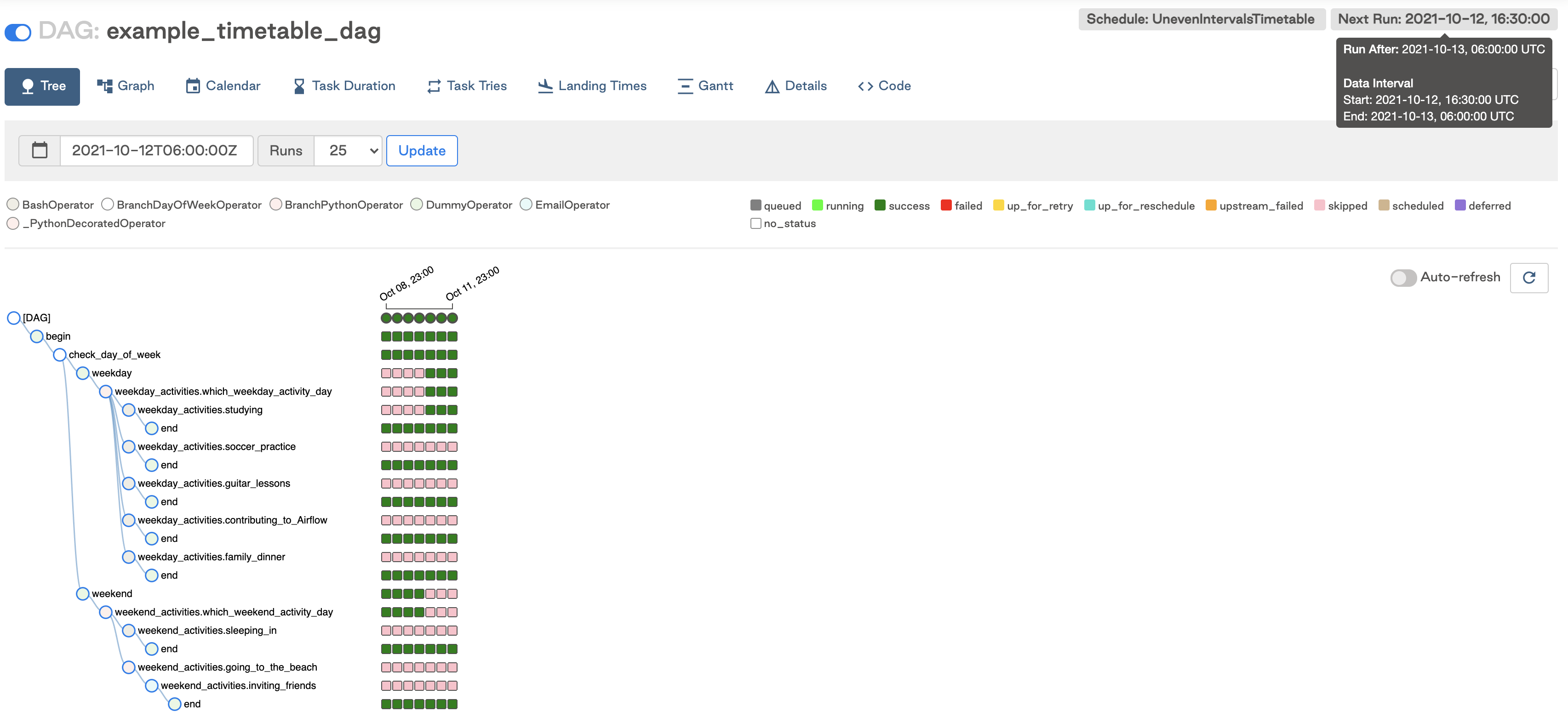If we run the DAG manually after 16:30 but before midnight, we can see the data interval for the triggered run was between 6:00 and 16:30 that day as expected.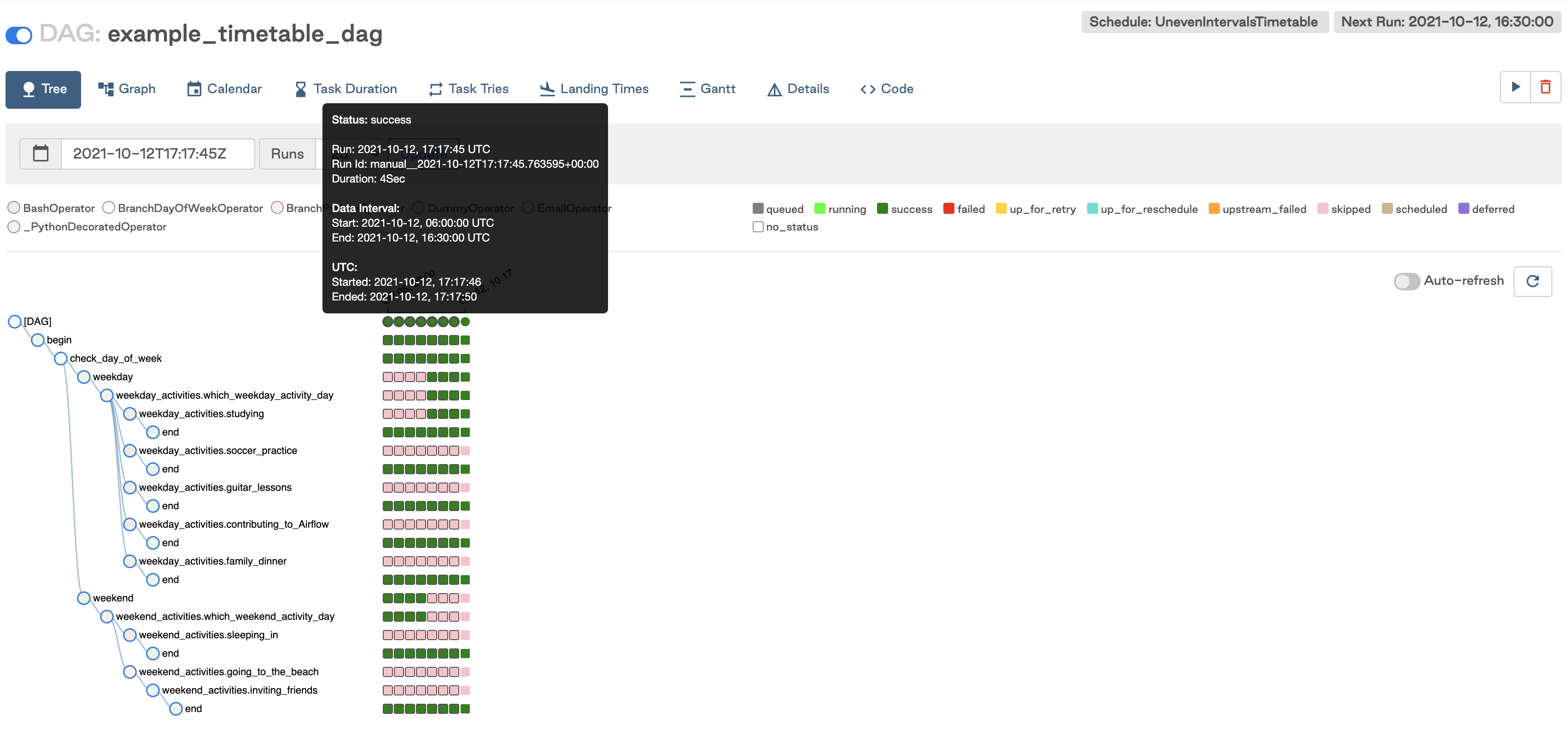This is a simple timetable that could easily be adjusted to suit other use cases. In general, timetables are completely customizable as long as the methods above are implemented.

Note: Be careful when implementing your timetable logic that your `next_dagrun_info` method does not return a `data_interval_start` that is earlier than your DAG’s `start_date`. This will result in tasks not being executed for that DAG run.

### Current Limitations

There are some limitations to keep in mind when implementing custom timetables:

• Timetable methods should return the same result every time they are called (e.g. avoid things like HTTP requests). They are not designed to implement event-based triggering.
• Timetables are parsed by the scheduler when creating DAG runs, so avoid slow or lengthy code that could impact Airflow performance.

## Dataset driven scheduling

Airflow 2.4 introduced the concept of datasets and data-driven DAG dependencies. You can now make Airflow detect when a task in a DAG updates a data object. Using that awareness, other DAGs can be scheduled depending on updates to these datasets. To create a dataset-based schedule, you simply pass the names of the datasets as a list to the `schedule` parameter.

``````dataset1 = Dataset(f"{DATASETS_PATH}/dataset_1.txt")
dataset2 = Dataset(f"{DATASETS_PATH}/dataset_2.txt")

with DAG(
dag_id='dataset_dependent_example_dag',
catchup=False,
start_date=datetime(2022, 8, 1),
schedule=[dataset1, dataset2],
tags=['consumes', 'dataset-scheduled'],
) as dag:``````

This DAG runs only when both `dataset1` and `dataset2` have been updated. These updates can occur by tasks in different DAGs as long as they are located in the same Airflow environment.

In the Airflow UI, the DAG now has a schedule of Dataset and the Next Run column shows how many datasets the DAG depends on and how many of them have been updated.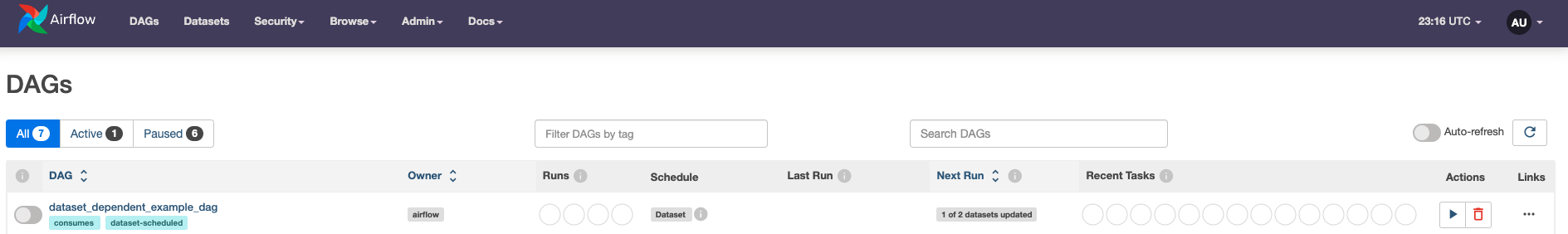To learn more about datasets and data driven scheduling, check out the Datasets and Data Driven Scheduling in Airflow guide.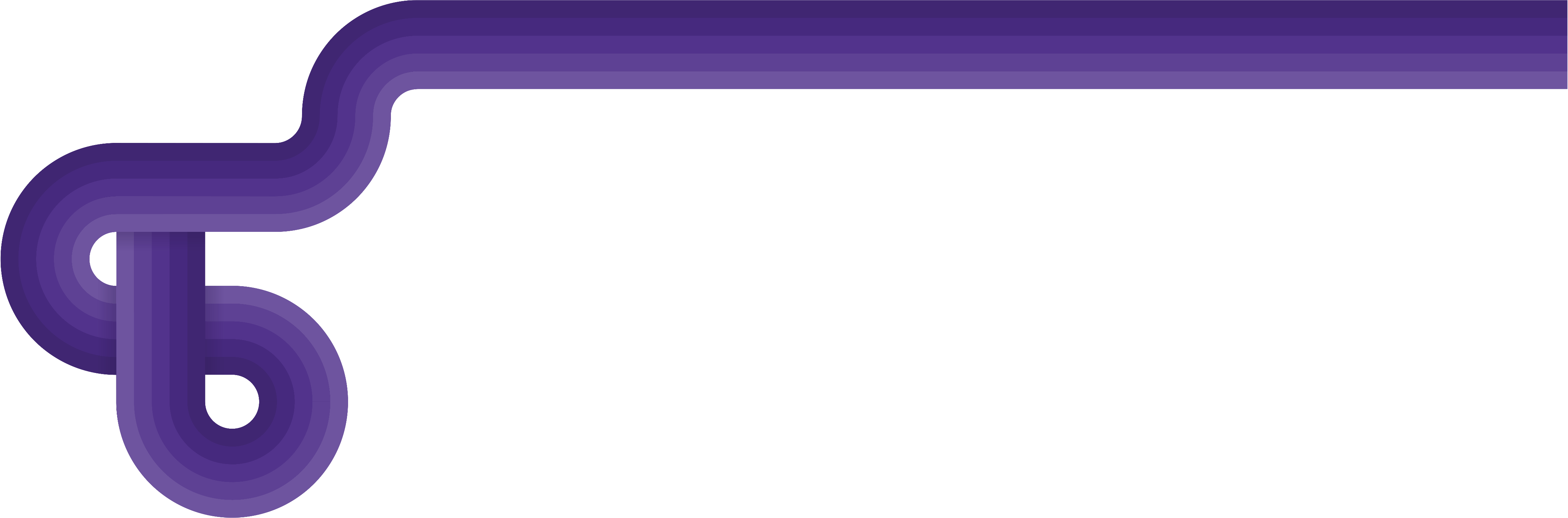Start building your next-generation data platform with Astro.